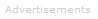# Definition of faraday

What is the faraday?

One faraday of electric charge corresponds to the charge on a mole of electrons, that is 6.022 x 1023 electrons; hence one faraday of charge is equal to 96,485.33 coulombs.

1 faraday = 96,485.33 C
What is the Faraday Constant?

We can write the Faraday constant F:

F = 96,485.33 C mol-1Search the Dictionary for More Terms